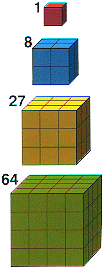# Cubes

The first six cube numbers are –
1, 8, 27, 64, 125, 216

They are equal to –
1 × 1 × 1, 2 × 2 × 2, 3 × 3 × 3, 4 × 4 × 4, 5 × 5 × 5, 6 × 6 × 6
– which can be written more neatly as –
13, 23, 33, 43, 53, 63
– where the small 3 means cubed.

The cube of 5 is 125, and working backwards, we say the cube root of 125 is 5.

There is a connection between cube numbers and cube shapes. If you use cube shaped bricks to build a larger cube, the number of bricks you need is a cube number. For example, if you wanted to build an 8cm cube using 1cm cube bricks you would need 512 bricks which is the cube of 8.The first four cubes.

Based on the book Numbers: Facts, Figures & Fiction.